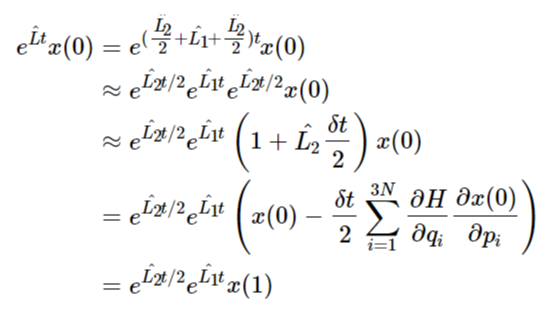# 从刘维尔方程到Velocity-Verlet算法本文延续历史上分子动力学模拟演化算法的发展顺序，分别讲述了Verlet、LeapFrog和Velocity-Verlet三个算法的形式，并且结合刘维尔方程，推导了Velocity-Verlet算法中的三个演化步骤的内在含义。三种不同的演化算法，都有不同的初始依赖，而对于计算过程而言，越多的初始依赖，就会涉及到越多的参数存储问题。一个好的演化算法，只需要依赖于少量的参数，而具备较高的精度。

# Verlet算法

$r(t+\delta t)=r(t)+v(t)\delta t+\frac{F(t)}{2m}\delta t^2\\ r(t-\delta t)=r(t)-v(t)\delta t+\frac{F(t)}{2m}\delta t^2$

$r(t+\delta t)=2r(t)-r(t-\delta t)+\frac{F(t)}{m}\delta t^2+O(\delta t^4)$

$v(t)=\frac{r(t+\delta t)-r(t-\delta t)}{2\delta t}+O(\delta t^2)$

1. 第一步的更新，没有上上一步的信息；
2. 在算法执行的过程中，每次迭代不仅仅要存储上一步的坐标位置，还需要额外存储上上一步的位置，更新较为麻烦，也会占据额外的空间。

# Leap-Frog算法

$r(t+\delta t)=r(t)+v(t+\frac{\delta t}{2})\delta t$

$v(t+\frac{\delta t}{2})=v(t-\frac{\delta t}{2})+\frac{F(t)\delta t}{m}=v(t-\frac{\delta t}{2})-\frac{\partial V}{\partial r(t)}\cdot\frac{\delta t}{m}$

$v(t)=\frac{v(t+\frac{\delta t}{2})-v(t-\frac{\delta t}{2})}{2}$

# 刘维尔方程与Velocity Verlet

$\frac{d\rho(p,q,t)}{dt}=\frac{\partial\rho}{\partial t}+\hat{L}\rho=0$

$\hat{L}=\hat{L_1}+\hat{L_2}=\sum_{i=1}^{3N}\left(\frac{\partial H}{\partial p_i}\frac{\partial}{\partial q_i}-\frac{\partial H}{\partial q_i}\frac{\partial}{\partial p_i}\right)$

$\hat{L_1}=\sum_{i=1}^{3N}\frac{\partial H}{\partial p_i}\frac{\partial}{\partial q_i}\\ \hat{L_2}=-\sum_{i=1}^{3N}\frac{\partial H}{\partial q_i}\frac{\partial}{\partial p_i}$

$N=\int\rho(p,q,t)dqdp$

## Trotter-Suzuki分解

$e^{\sum_{j=1}^{m}H_jt}=\prod_{j=1}^{m}e^{H_jt}+O(m^2t^2)$

\begin{align} e^{\hat{L}t}x(0)&=e^{(\frac{\hat{L_2}}{2}+\hat{L_1}+\frac{\hat{L_2}}{2})t}x(0)\\ &\approx e^{\hat{L_2}t/2}e^{\hat{L_1}t}e^{\hat{L_2}t/2}x(0)\\ &\approx e^{\hat{L_2}t/2}e^{\hat{L_1}t}\left(1+\hat{L_2}\frac{\delta t}{2}\right)x(0)\\ &=e^{\hat{L_2}t/2}e^{\hat{L_1}t}\left(x(0)-\frac{\delta t}{2}\sum_{i=1}^{3N}\frac{\partial H}{\partial q_i}\frac{\partial x(0)}{\partial p_i}\right)\\ &=e^{\hat{L_2}t/2}e^{\hat{L_1}t}x(1) \end{align}

\begin{align} e^{\hat{L}t}x(0)&\approx e^{\hat{L_2}t/2}e^{\hat{L_1}t}x(1)\\ &=e^{\hat{L_2}t/2}\left(x(1)+\delta t\sum_{i=1}^{3N}\frac{\partial H}{\partial p_i}\frac{\partial x(1)}{\partial q_i}\right)\\ &=e^{\hat{L_2}t/2}x(2) \end{align}

\begin{align} e^{\hat{L}t}x(0)&\approx e^{\hat{L_2}t/2}x(2)\\ &=x(2)-\frac{\delta t}{2}\sum_{i=1}^{3N}\frac{\partial H}{\partial q_i}\frac{\partial x(2)}{\partial p_i}\\ &=x(3) \end{align}

## Velocity Verlet算法

$v(t+\frac{\delta t}{2})=v(t)+\frac{F(t)}{m}\frac{\delta t}{2}+O(\delta t^3)$

$r(t+\delta t)=r(t)+v(t+\frac{\delta t}{2})\delta t+O(\delta t^4)$

$v(t+\delta t)=v(t+\frac{\delta t}{2})+\frac{F(t+\delta t)}{m}\frac{\delta t}{2}+O(\delta t^2)$

# 版权声明

2 total views,  1 views today